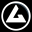• Register

## Delta G

### Evols | Early Access May 30, 2019

Delta-G is an online multiplayer FPS. Its specifity is that you can change the gravity direction, so you can walk on walls and roofs. The core idea of the game is the idea of freedom of movement. You also can use a hook, to move faster, and fly in the air.

How we used quaternions to change gravity

To have a multi-gravity character movement, we don't just use vectors, but more advanced mathematical concepts, such as quaternions

Posted by on

The core feature of Delta-G is that you can change the gravity direction. While this is a good idea (or at least we hope it is), it's also a nightmare, coding wise. Why is that? Because most modern game engines are designed for classic, -Z gravity, especially, Unreal Engine 4, the engine we're using. Thus, we can't use UE4's built-in character movement system, and we had to create ours.

### At the beginning, gravity as a vector

Our naïve approach to gravity was... well, to just change the direction of the gravity vector, so insteag of being (0,0,-g), it would be some other value. While it worked great for simple cases, it was not powerful enough to make a complete character movement system, because movement had to be adapted to this new gravity, view had to be rotated, and a simple vector just can't represent all this data.

To make this vector-based approach more powerful, we chose to compute the rotation that the gravity change implied (in Euler angles, or Rotator in UE4, which do a rotation around the Z axis, then the Y axis, then the X axis). Let's say, if you've changed the gravity from being -Z to +Y, then you've rotated 90° around the X axis. Now, how do we compute that? Well, to find the axis of rotation, you just have to do the cross product of the gravity vectors (oldGravity ^ newGravity), and to get the angle, you compute the arccosine of the dot product of the gravity vectors (acos(oldGravity . newGravity)).

### Enters the quaternions

You may have seen that the axis around which we rotate might not be a plain axis (it could be (0.707, 0.707, 0)), and translating this to Euler angles is not a cool thing to do, so instead, we decided to use quaternions. What are quaternions? Just another way of thinking of rotations: they represent the rotation by the axis of rotation, and the angle, so we can rotate around an arbitrary axis. Awesome, isn't it? We can just the data we computed earlier !

Then, we just had to rotate the axes of movement of the character by the obtained quaternion, and to rotate the view by the quaternion too. While this kinda worked, we figured out we needed something more simple, more unified. So instead of computing a quaternion at each gravity change, with the computation error it brought, why don't we just represent the gravity as a quaternion, instead of a vector? I mean, the gravity is anyway just a combination of quaternions, that rotate the character with each gravity change.

### To a quaternion-only architecture

This is how we came to completely remove the gravity vector, and go with a full-quaternion architecure. The gravity quaternion was indeed how you have to rotate the "character as if it were on a classic floor" to get the "character as he really is". And for each gravity change, you just had to rotate the gravity quaternion by a given quaternion, called the delta gravity quaternion.

Also, the view-computhing and movement-computing algorithm changed. The base view rotation is still handled as Euler angles (when you move the mouse down, the Y value of the base view rotation increases, and when you move it right, the Z value of the base view rotation increases), but before being applied to the actual camera, it's multiplied by the gravity quaternion. Same thing for the movement input: before being applied to the character, it's rotated by the gravity quaternion.

All this is permitted because quaternions multiplication works like matrix multiplication: when you multiply a quaternion by another, it's just as if the 2nd quaternion's rotation was made in the 1st quaternion's coordinates system.

### End notes

Of course, using quaternions instead of Euler angles decreased performances, but not enough to impact framerate.

Thanks for reading ! I hope you now know a little bit more about Delta-G's architecture !

Only registered members can share their thoughts. So come on! Join the community today (totally free - or sign in with your social account on the right) and join in the conversation.

Profile
##### IconNews